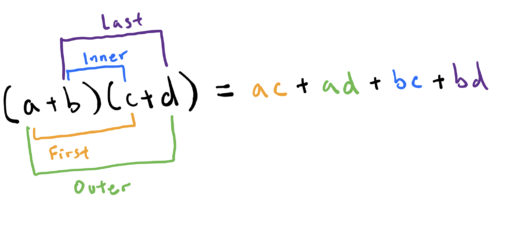In order to solve systems of equations, we need to understand three concepts: variables, how to manipulate equations, and the distributive law.

Variables

Variables are letters that stand in for numbers. Often, we will use the lettersas variables. We can use equations to assign a specific value to a certain variable. For example,means that the variableis equal to the number.

We use variables to capture certain relationships between quantities when we do not know the specific values involved. For example, I could tell you that my cell phone is worth twice as much as my laptop (and this is true). Without variables, we would have no way to represent this mathematically, since we do not know the actual value of either my laptop or cell phone. But with variables, we can say:the value of my laptop,the value of my cell phone. Then, we can represent this information mathematically as:Thus, by using variables, we can capture relationships between unknown values.

Manipulating Equations

The second idea we need to understand is how to manipulate equations. Often, we are given a complicated equation like:And we want to turn it into a simpler equation, likeNow, the key is that we are allowed to anything we like to one side of the equation provided we do it to the other side as well. So, for example, we can take our initial equationAnd we can subtract 8 from both sides to get:And we can divide by 3, provided we do it to both sides, to get:So now we know what the value ofis, namely 3.

The reason why this works is that in an equation, you are saying that the left hand side is equal to the right hand side. In other words, that the left hand side represents the very same number as the right hand side. So in our equation,We are saying thatis just another way of writing the number 17. So of course we can do anything we like to both sides and the resulting numbers will still be equal, since we are doing the same thing to the same numbers.

Remember, though, that we still cannot do mathematically illicit operations, like dividing by 0, even if we do them to both sides.

Distributive Law

Finally, we need to understand how the distributive law works. It is a rule for how multiplication and addition interact and it states:An example may help in seeing that the distributive law is, in fact, true:

We know that, and we know thatbecause operations in parenthesis are done before any other operations. Thus, we know thatAnd by the distributive law,So it is true thatsinceand(and of course).

Now, one very important application of the distributive law is used in factoring quadratic equations (as we discuss here). We will need to apply to the distributive law to:By distributing, we get:And in general, we will omit the multiplication symboland simply write:You may have also seen this formula called FOIL (First Outer Inner Last), a mnemonic meant to remind people of the four terms in our equation:However you choose to remember it, this formula is an important part of understanding how to factor equations.